# p e c ter n I ope l

• Slides: 48p e c ter n I ope l S ing h p a Gr m r o t. F e urc o s e al R on ne o l A d Stan cti u r t s In 1 1 8 P n o CE s n h o J h a r o b De Let’s Get Started! Click Here!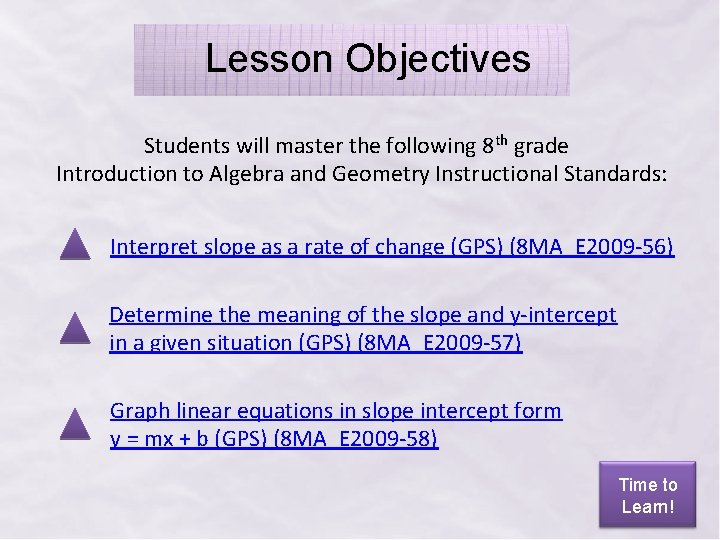Lesson Objectives Students will master the following 8 th grade Introduction to Algebra and Geometry Instructional Standards: Interpret slope as a rate of change (GPS) (8 MA_E 2009 -56) Determine the meaning of the slope and y-intercept in a given situation (GPS) (8 MA_E 2009 -57) Graph linear equations in slope intercept form y = mx + b (GPS) (8 MA_E 2009 -58) Time to Learn!F What do you need to know ! t irs about slope-intercept form? Click on the boxes below to explore each definition! Definition of Slope Definition of Intercepts Definition of Slope-Intercept Form Second! Let’s Graph!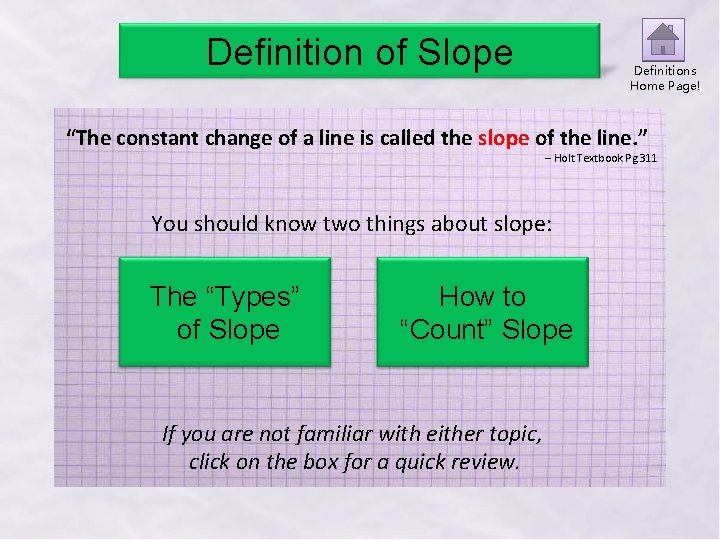Definition of Slope Definitions Home Page! “The constant change of a line is called the slope of the line. ” – Holt Textbook Pg 311 You should know two things about slope: The “Types” of Slope How to “Count” Slope If you are not familiar with either topic, click on the box for a quick review.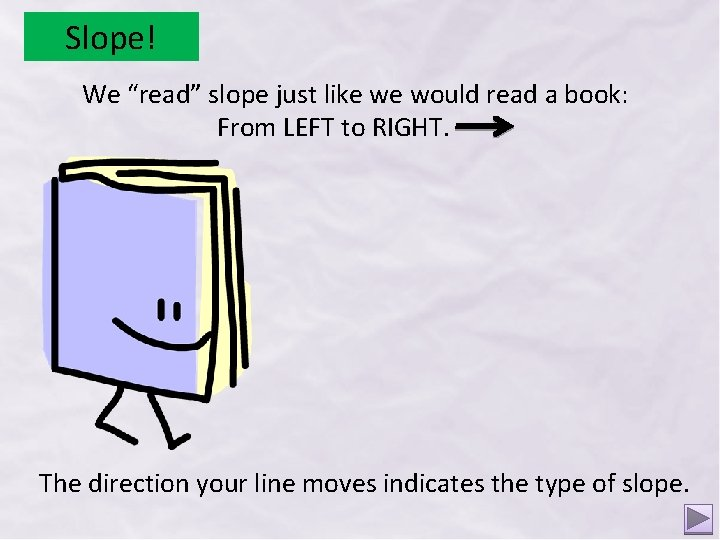Slope! We “read” slope just like we would read a book: From LEFT to RIGHT. The direction your line moves indicates the type of slope.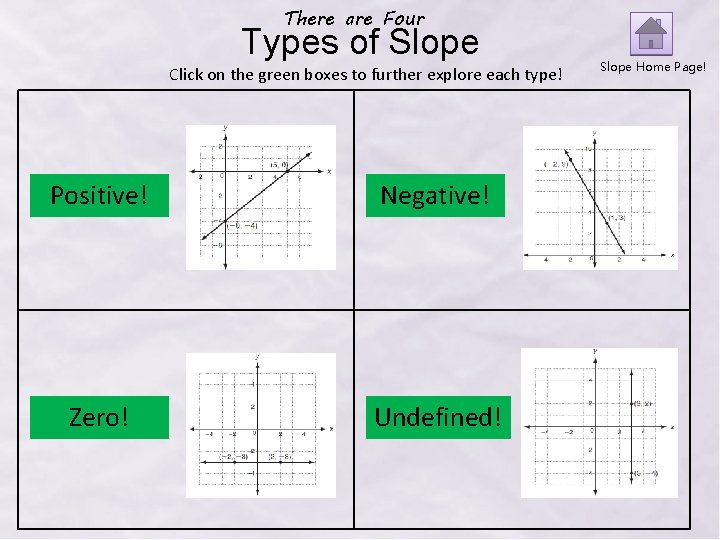There are Four Types of Slope Click on the green boxes to further explore each type! Positive! Negative! Zero! Undefined! Slope Home Page!Positive Slope! With POSITIVE slope, the line rises from left to right. It does not matter WHERE the line is located on the coordinate plane, but rather the direction. Often, we think of positive slope as a line going UP. We can see slope in real life! Can you see the POSITIVE slope in the picture above? (click on the picture to check your answer)Negative Slope! With NEGATIVE slope, the line falls from left to right. Often, we think of negative slope as a line going DOWN. Check out the picture to the left! Can you find the NEGATIVE slope? (click on the picture to check your answer)Zero Slope! Zero slope always looks like a HORIZONTAL line. An easy way to remember this is by visualizing a person walking on your line. The person has ZERO slope because they do not go up or down. Click on the graph to see this!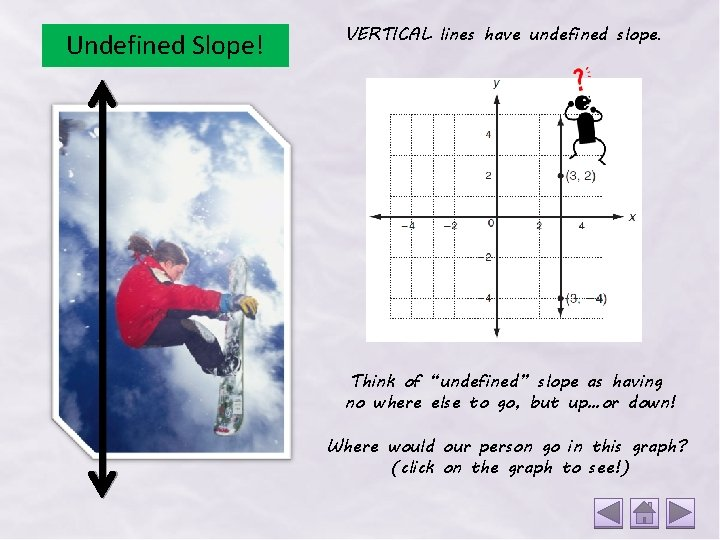Undefined Slope! VERTICAL lines have undefined slope. Think of “undefined” slope as having no where else to go, but up…or down! Where would our person go in this graph? (click on the graph to see!)Let’s Practice! Tell whether the slope of each line is positive, negative, zero, or undefined. Click on the graph to check your answer! Positive Negative Zero The graph rises from left to right. The graph falls from left to right. The graph is a horizontal line. Negative Undefined The graph falls from left to right. The graph is a vertical line. Positive The graph rises from left to right.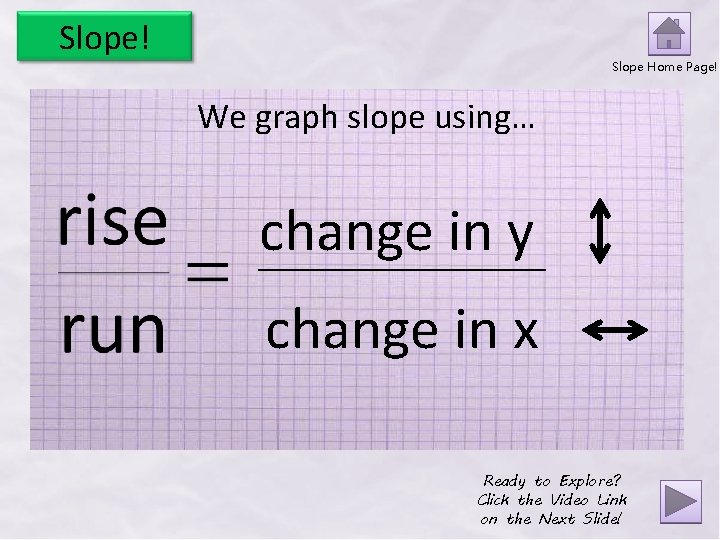Slope! Slope Home Page! We graph slope using… change in y change in x Ready to Explore? Click the Video Link on the Next Slide!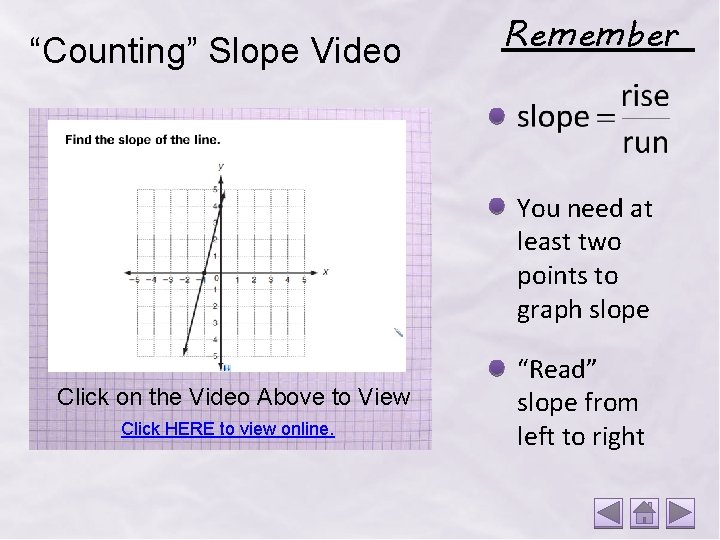“Counting” Slope Video Remember You need at least two points to graph slope Click on the Video Above to View Click HERE to view online. “Read” slope from left to right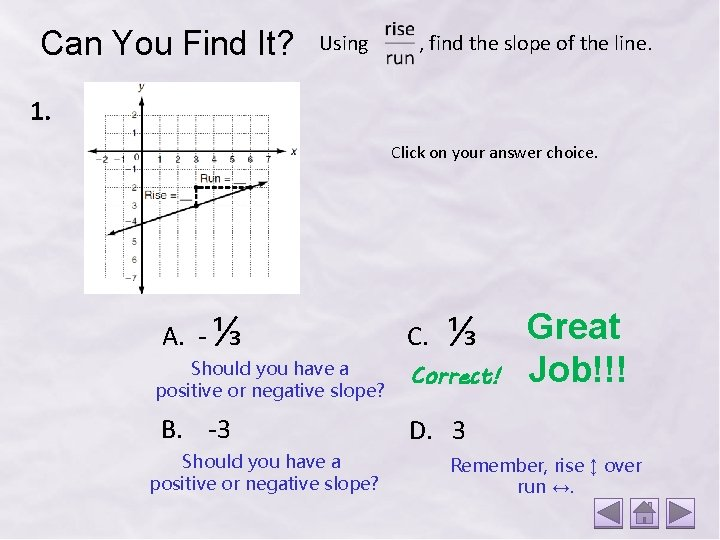Can You Find It? Using , find the slope of the line. 1. Click on your answer choice. A. - ⅓ Should you have a positive or negative slope? B. -3 Should you have a positive or negative slope? C. ⅓ Correct! Great Job!!! D. 3 Remember, rise ↕ over run ↔.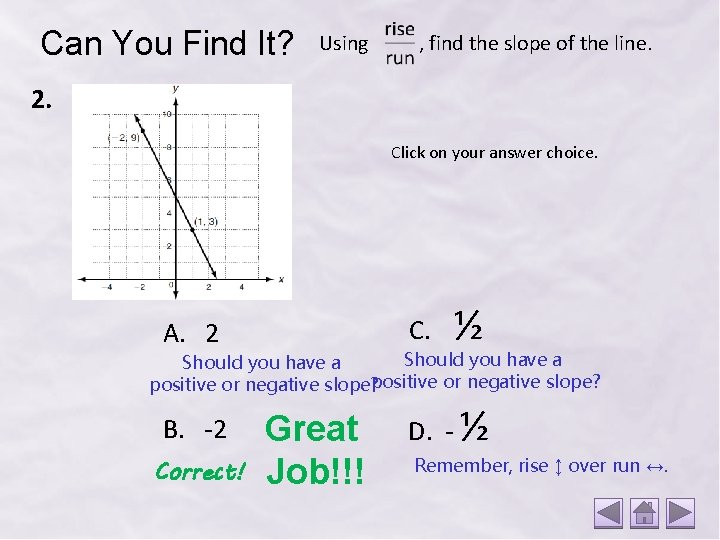Can You Find It? Using , find the slope of the line. 2. Click on your answer choice. C. A. 2 ½ Should you have a positive or negative slope? B. -2 Correct! Great Job!!! D. - ½ Remember, rise ↕ over run ↔.Need more practice? Using algebra. help, look at each graph and enter the appropriate slope. Click on “Check Worksheet” at the bottom of the page to check your work! Practice Here! Ready to move on? Back to Definitions!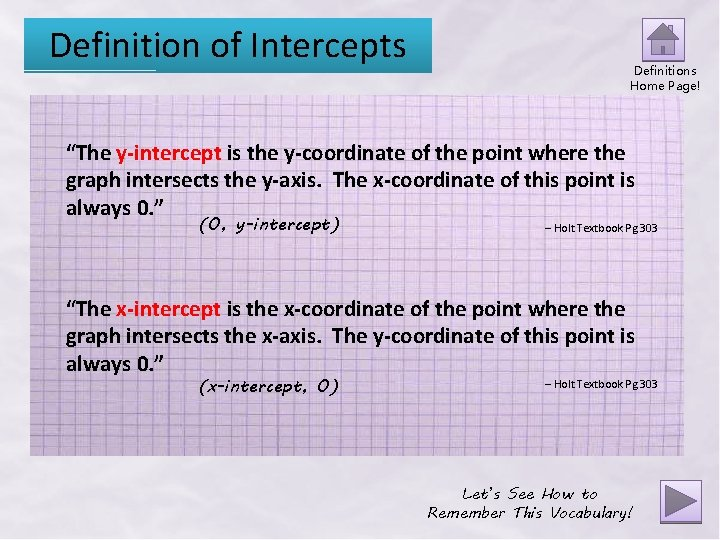Definition of Intercepts Definitions Home Page! “The y-intercept is the y-coordinate of the point where the graph intersects the y-axis. The x-coordinate of this point is always 0. ” (0, y-intercept) – Holt Textbook Pg 303 “The x-intercept is the x-coordinate of the point where the graph intersects the x-axis. The y-coordinate of this point is always 0. ” (x-intercept, 0) – Holt Textbook Pg 303 Let’s See How to Remember This Vocabulary!When you hear “intercepts, ” think: Football! http: //espn. go. com/video/clip? id=5667192 Think of your line like the “path” Denard Robinson wanted his football to be thrown. The y-intercept represents the point where the line crosses the y-axis. For our story, this is where Chris L. Rucker “intercepts” the ball. Click on the Player to Hear a Story about the Video! The x-intercept represents the point where the line crosses the x-axis. For our story, let’s suppose Michigan State “intercepts” the ball again at the x-axis.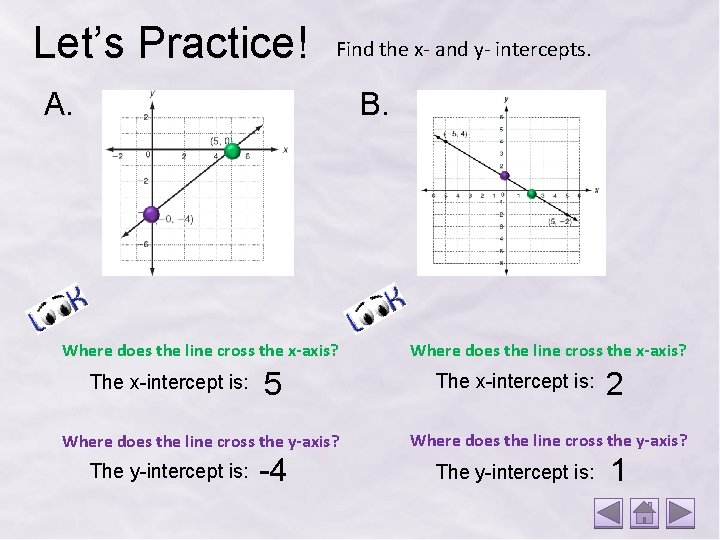Let’s Practice! Find the x- and y- intercepts. A. B. Where does the line cross the x-axis? The x-intercept is: 5 Where does the line cross the y-axis? The y-intercept is: -4 Where does the line cross the x-axis? The x-intercept is: 2 Where does the line cross the y-axis? The y-intercept is: 1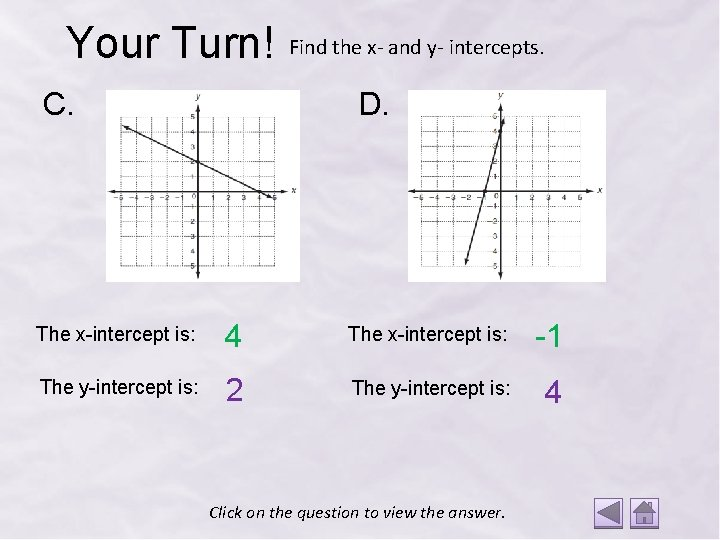Your Turn! C. Find the x- and y- intercepts. D. The x-intercept is: 4 The x-intercept is: -1 The y-intercept is: 2 The y-intercept is: 4 Click on the question to view the answer.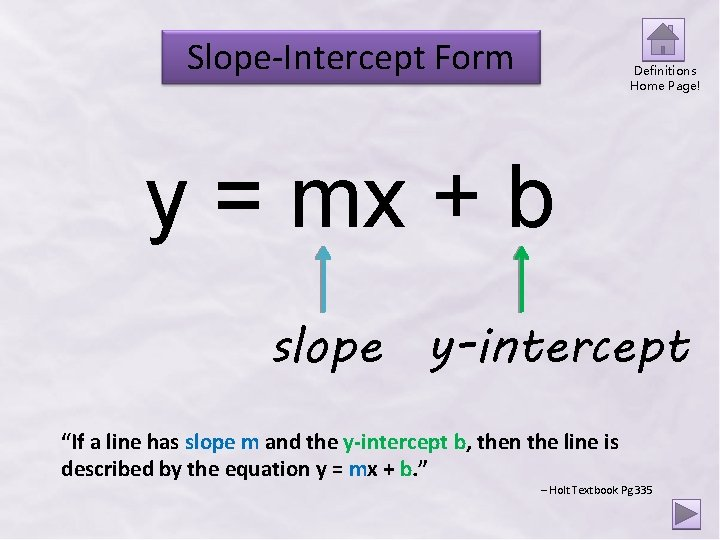Slope-Intercept Form Definitions Home Page! y = mx + b slope y-intercept “If a line has slope m and the y-intercept b, then the line is described by the equation y = mx + b. ” – Holt Textbook Pg 335Slope-Intercept Form Can you recognize slope-intercept form? Let’s Play… Slope-Intercept Form or Not!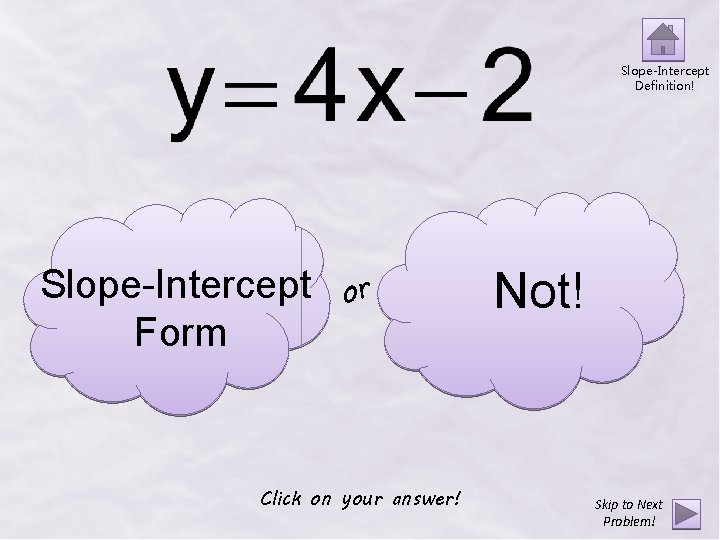Slope-Intercept Definition! Slope-Intercept Form or Click on your answer! Not! Skip to Next Problem!Correct! What is the slope? 4 What is the y-intercept? Click on the question to view the answer. -2 Next Problem!Try Again! Remember… y = mx + b slope y-intercept Go Back!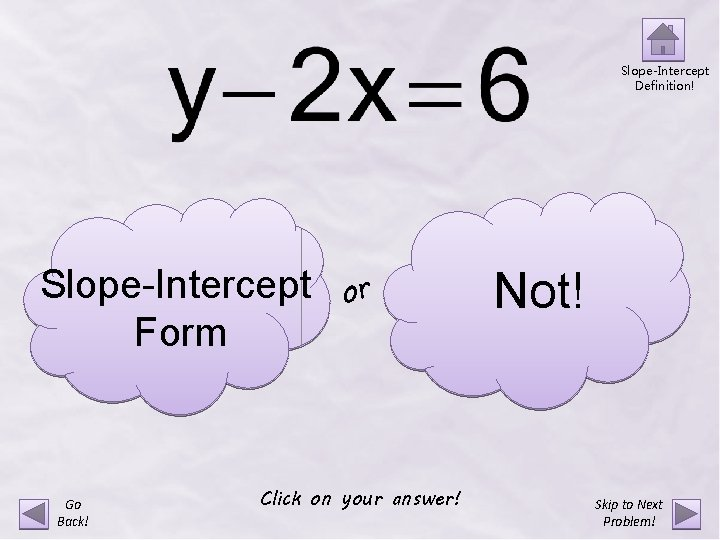Slope-Intercept Definition! Slope-Intercept Form Go Back! or Click on your answer! Not! Skip to Next Problem!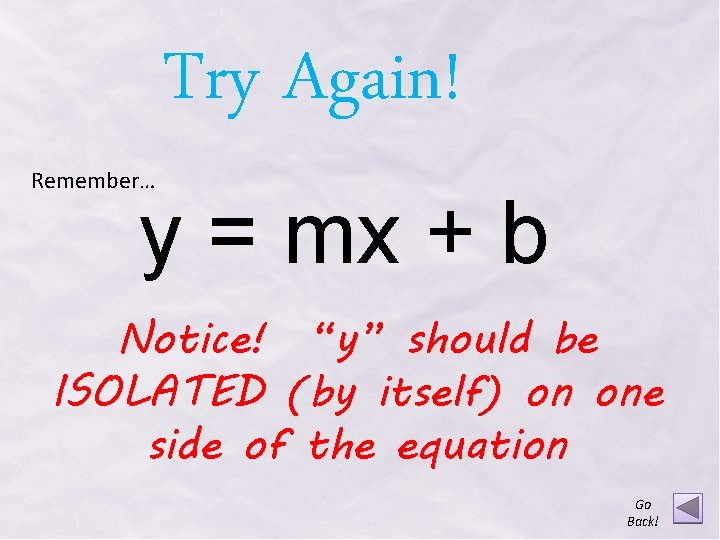Try Again! Remember… y = mx + b Notice! “y” should be ISOLATED (by itself) on one side of the equation Go Back!Correct! Is NOT in slope-intercept form! Check out how to re-write this equation in slopeintercept form!+ 2 x y = 2 x + 6 The slope is 2. The y-intercept is 6. Next Problem!Slope-Intercept Definition! Slope-Intercept Form Go Back! or Click on your answer! Not!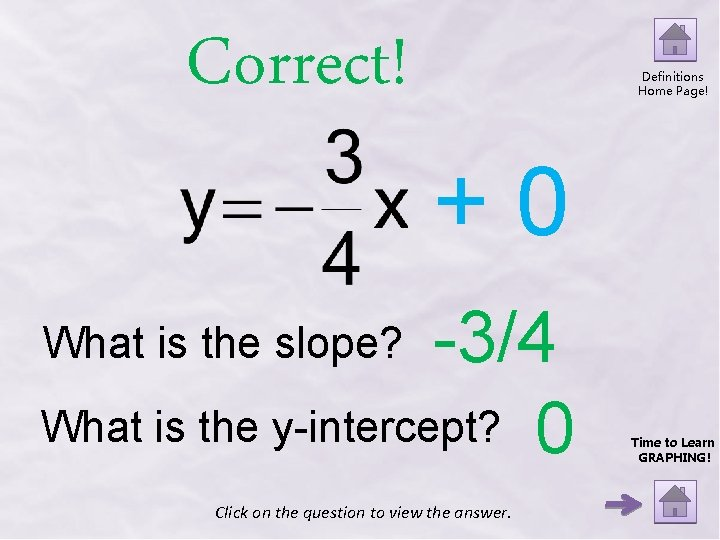Correct! Definitions Home Page! +0 -3/4 What is the y-intercept? 0 What is the slope? Click on the question to view the answer. Time to Learn GRAPHING!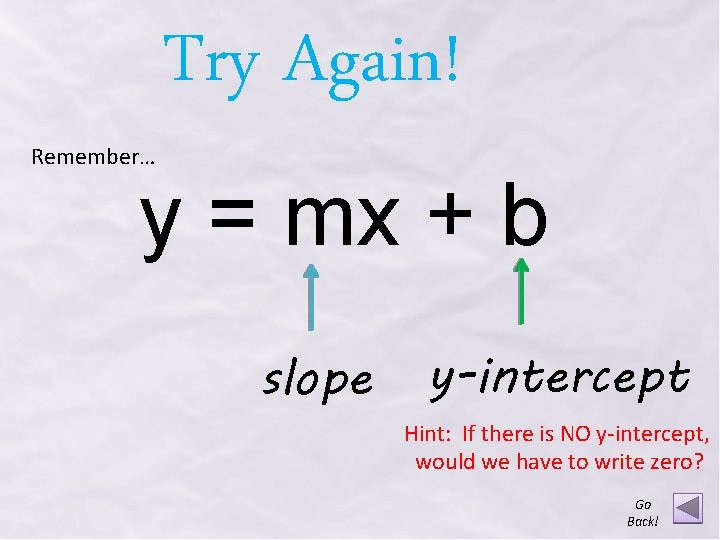Remember… Try Again! y = mx + b slope y-intercept Hint: If there is NO y-intercept, would we have to write zero? Go Back!Graphing Slope-Intercept Form ! t irs F Graphing Slope. Intercept Form ! d on c e S Matching Equations to Graphs Back to Definitions Home Page!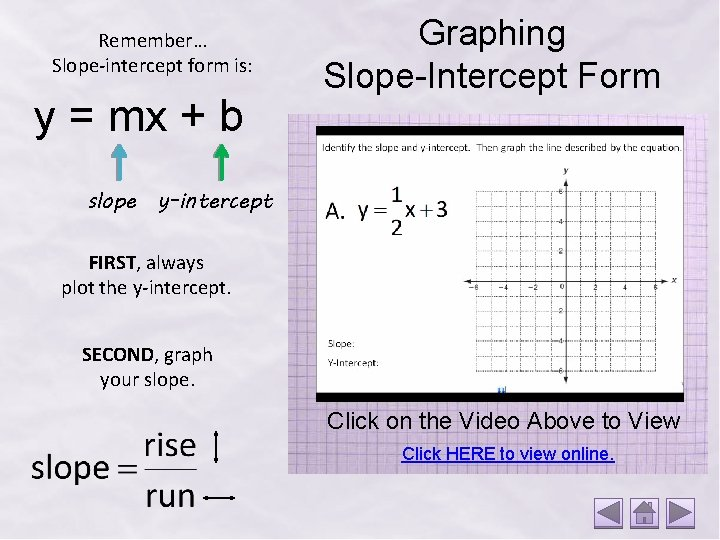Remember… Slope-intercept form is: y = mx + b Graphing Slope-Intercept Form slope y-intercept FIRST, always plot the y-intercept. SECOND, graph your slope. Click on the Video Above to View Click HERE to view online.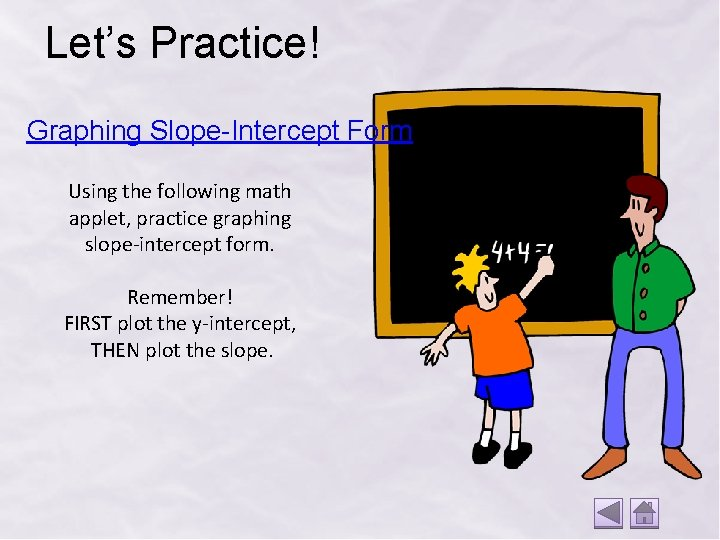Let’s Practice! Graphing Slope-Intercept Form Using the following math applet, practice graphing slope-intercept form. Remember! FIRST plot the y-intercept, THEN plot the slope.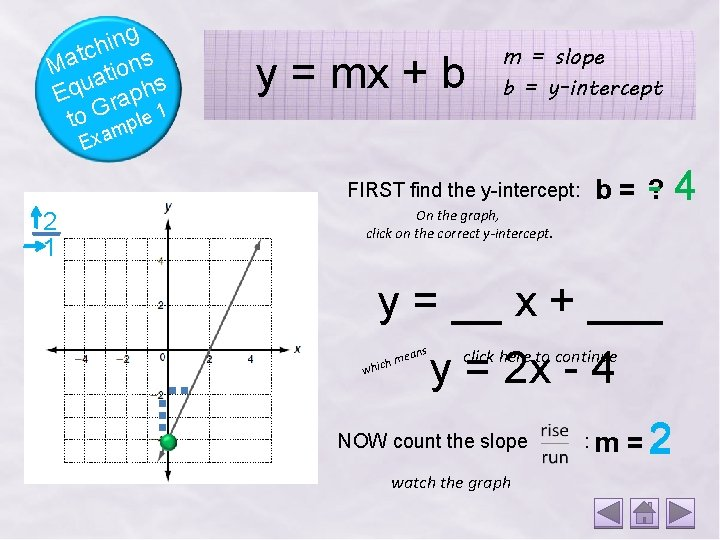g n i h tc ns a M io t a Equ raphs to G le 1 y = mx + b m = slope b = y-intercept p m a Ex FIRST find the y-intercept: 2 1 On the graph, click on the correct y-intercept. b= ? - y = __ x + ___ ans h me whic y = 2 x - 4 click here to continue NOW count the slope watch the graph : m =2 4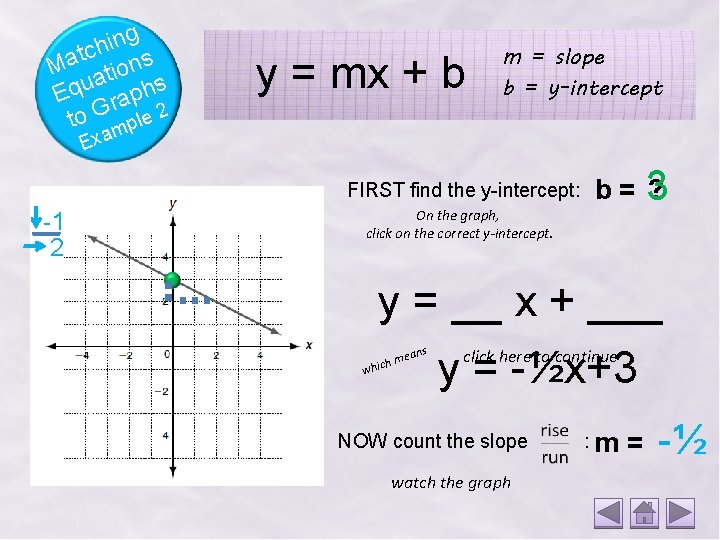g n i h tc ns a M io t a Equ raphs to G le 2 y = mx + b m = slope b = y-intercept p m a Ex FIRST find the y-intercept: -1 2 On the graph, click on the correct y-intercept. b=3 ? y = __ x + ___ ans h me whic y = -½x+3 click here to continue NOW count the slope watch the graph : m = -½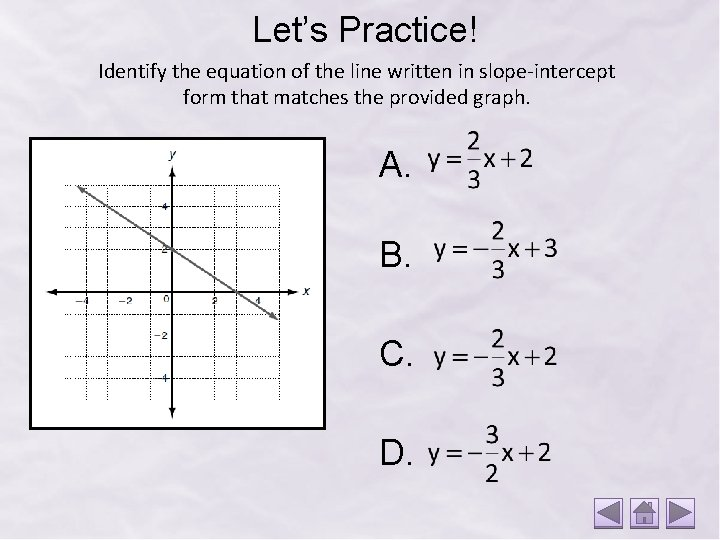Let’s Practice! Identify the equation of the line written in slope-intercept form that matches the provided graph. A. B. C. D.Try Again! Check Your Slope! Should your slope be Positive or Negative? Did you mix up ? Go Back!Try Again! Check Your Intercep y = mx + b Make sure you picked the “Y” intercept and not the “X” intercept! Go Back!Correct! Next Problem!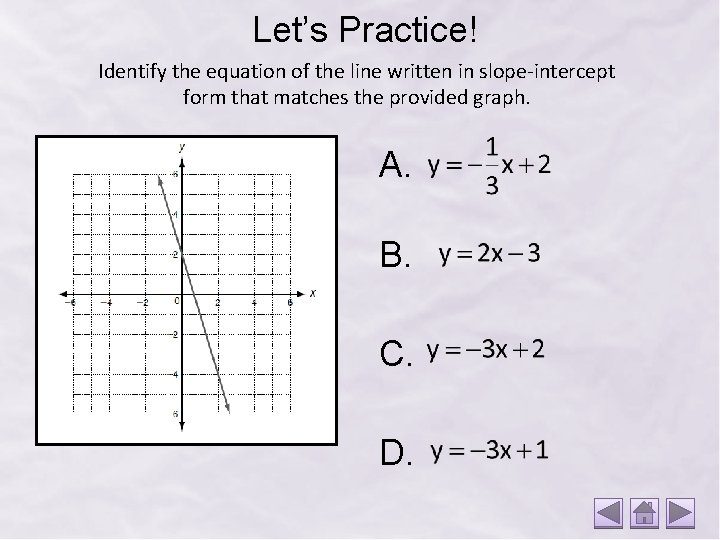Let’s Practice! Identify the equation of the line written in slope-intercept form that matches the provided graph. A. B. C. D.Try Again! Check Your Slope! Should your slope be Positive or Negative? Did you mix up ? Go Back!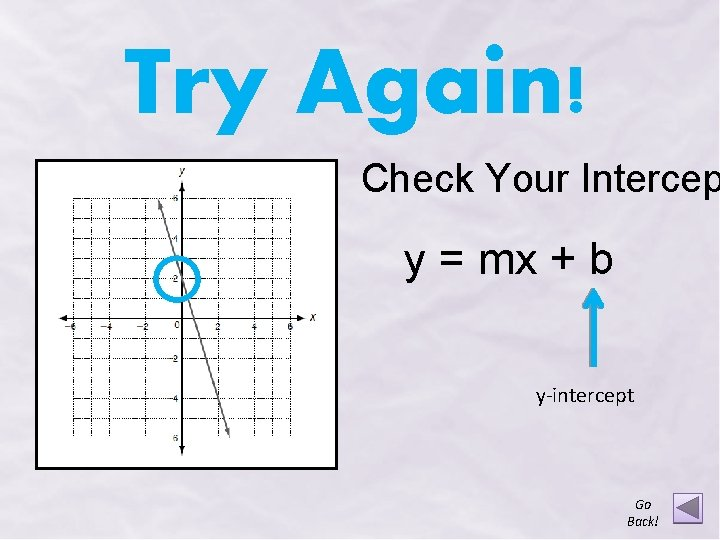Try Again! Check Your Intercep y = mx + b y-intercept Go Back!Correct! Next Problem!Final Challenge! Write the equation of the line for each provided graph. To check you answer, click on the graph! Need a Hint? Click HERE to view the equations that need to be matched to the graphs!You Did It! You have successfully completed the “Graphing Slope-Intercept Form Stand-Alone Instructional Resource!” t a e Gr ! ! ! b o JResources Author: Deborah Johnson Created in: Microsoft Power. Point Definitions and math images: Holt Algebra 1 Textbook Holt, Rinehart and Winston Publications Clip art: Microsoft Power. Point Sound: Microsoft Power. Point & Soungle Websites: http: //www. algebrahelp. com/worksheets/view/graphing/slope. quiz http: //espn. go. com/video/clip? id=5667192 http: //www. ltcconline. net/greenl/java/Basic. Algebra/Line. Graph. htm Videos: Created by Deborah Johnson using Interwrite Learning